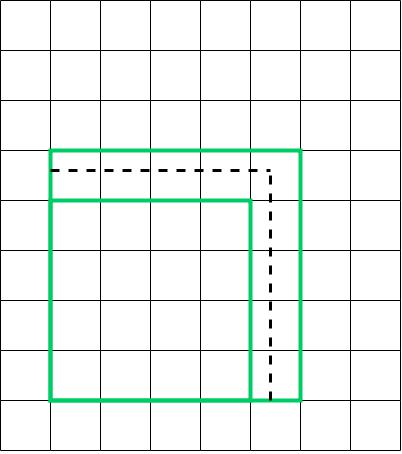# Determine square roots of rational numbers#### Everything You Need in One Place

Homework problems? Exam preparation? Trying to grasp a concept or just brushing up the basics? Our extensive help & practice library have got you covered.#### Learn and Practice With Ease

Our proven video lessons ease you through problems quickly, and you get tonnes of friendly practice on questions that trip students up on tests and finals.#### Instant and Unlimited Help

Our personalized learning platform enables you to instantly find the exact walkthrough to your specific type of question. Activate unlimited help now!

0/15
##### Examples
###### Lessons
1. Use the graph below to determine a rational number with a square root between 4 and 5.1. Use the side lengths below to estimate and calculate the area of each square.
1. 5.2 cm
2. 0.027 km
2. Find out if each of the following rational numbers is a perfect square.
1. $\frac{{81}}{{16}}$
2. 0.1
3. 0.01
4. $\frac{5}{{14}}$
3. Evaluate.
1. $\sqrt {361}$
2. $\sqrt {2209}$
3. $\sqrt {0.0169}$
4. $\sqrt {5.76}$
4. Calculate.
1. $\sqrt {56}$, to the nearest tenth
2. $\sqrt {3.7}$, to the nearest hundredth
3. $\sqrt {0.96}$ , to the nearest hundredth
4. $\sqrt {0.066}$ , to the nearest hundredth
0%
##### Practice
###### Topic Notes
In this section, we will look at how to evaluate a rational number by using square roots. We will also work on questions determining whether a rational number is a perfect square. We will also evaluate the square roots of rational numbers.